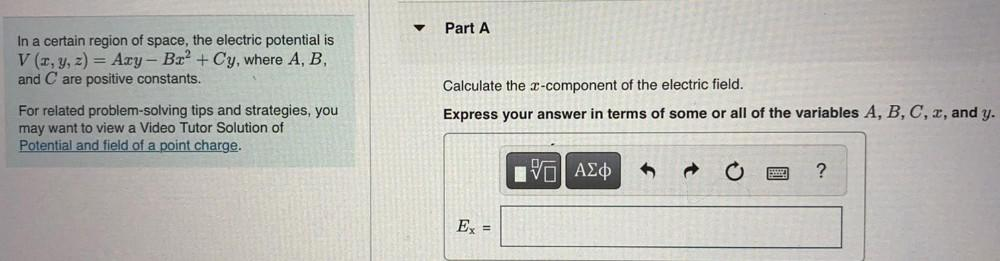Question:

# Part A In a certain region of space, the electric potential is V(x, y, z) = Azy - B.x2 + Cy, where A, B, and C are positive consPart A In a certain region of space, the electric potential is V(x, y, z) = Azy - B.x2 + Cy, where A, B, and C are positive constants. Calculate the x-component of the electric field. Express your answer in terms of some or all of the variables A, B, C, 2, and y. For related problem-solving tips and strategies, you may want to view a Video Tutor Solution of Potential and field of a point charge. V AED ? Ex =